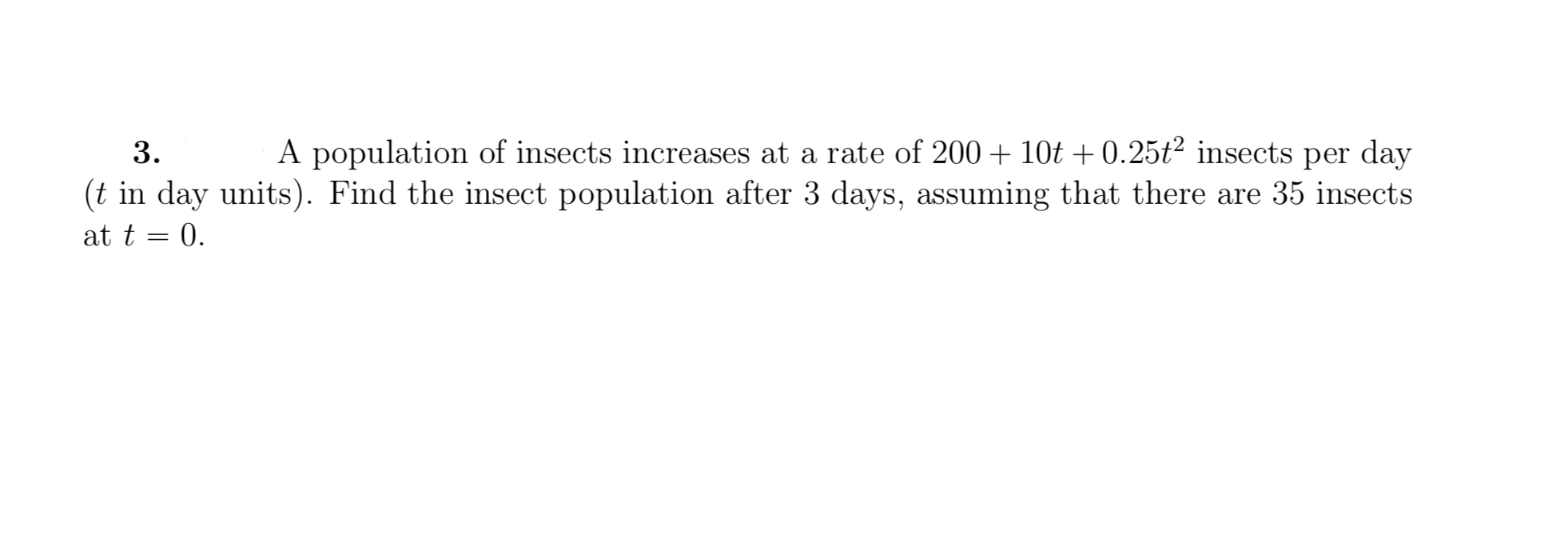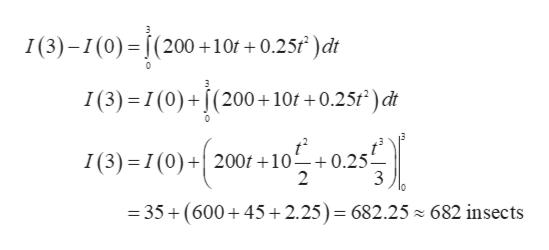# A population of insects increases at a rate of 20010t 0.25t2 insects per day3.(t in day units). Find the insect population after 3 days, assuming that there are 35 insectsat t 0

Question
170 viewshelp_outlineImage TranscriptioncloseA population of insects increases at a rate of 20010t 0.25t2 insects per day 3. (t in day units). Find the insect population after 3 days, assuming that there are 35 insects at t 0 fullscreen
check_circle

star
star
star
star
star
1 Rating
Step 1

It is given that initially there are 35 insects at t = 0. So, I (0) = 35.

Step 2

Let I (3) is the amount of insects after 3...help_outlineImage TranscriptioncloseI(3)-(0) (200 10f +0.25f )dt 0 I(3) 1(0)+(200+10f +0.25f)dt I(3) = I (0)+ 200t10 0.25 2 =35+(600+45 2.25)= 682.25 ~ 682 insects fullscreen

### Want to see the full answer?

See Solution

#### Want to see this answer and more?

Solutions are written by subject experts who are available 24/7. Questions are typically answered within 1 hour.*

See Solution
*Response times may vary by subject and question.
Tagged in

### Integration